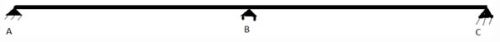Courses

# Test: Influence Lines For Statically Indeterminate Beams - 2

## 9 Questions MCQ Test Structural Analysis | Test: Influence Lines For Statically Indeterminate Beams - 2

Description
This mock test of Test: Influence Lines For Statically Indeterminate Beams - 2 for GATE helps you for every GATE entrance exam. This contains 9 Multiple Choice Questions for GATE Test: Influence Lines For Statically Indeterminate Beams - 2 (mcq) to study with solutions a complete question bank. The solved questions answers in this Test: Influence Lines For Statically Indeterminate Beams - 2 quiz give you a good mix of easy questions and tough questions. GATE students definitely take this Test: Influence Lines For Statically Indeterminate Beams - 2 exercise for a better result in the exam. You can find other Test: Influence Lines For Statically Indeterminate Beams - 2 extra questions, long questions & short questions for GATE on EduRev as well by searching above.
QUESTION: 1

### What will be the value of Rba?

Solution:

Explanation: Δba will be zero as it is roller supported.

QUESTION: 2

### What will be the value of Rca?

Solution:

Explanation: It will be the ration of Δca and Δaa. Δaa is 1944/EI and Δca is 1656/EI

QUESTION: 3

### What will be the value of Rda?

Solution:

Explanation: It will be the ration of Δda and Δaa. Δaa is 1944/EI and Δca is 936/EI. Join all these point for ILD.

QUESTION: 4

What will be the slope of ILD at point A?

Solution:

Explanation: Since point A is fixed joint, slope will always be zero there.

QUESTION: 5

What will be the shape of overall ILD?

Solution:

Explanation: ILD will be basically(approximately) a line passing through all above points but it will be a type of curve as slope near A will be zero and then slope will change.

QUESTION: 6

A and C is a pin support while B is roller support.
XL and XR are centroid of uniform loading on beams AB and BC respectively.
IL and IR are given.
LR and LL are length of beams.
AR and AL are areas of beams.Q. What is the change in slope at point B?

Solution:

Explanation: Since point B is roller support, there won’t be any change at of slope at point B.

QUESTION: 7

A and C is a pin support while B is roller support.
XL and XR are centroid of uniform loading on beams AB and BC respectively.
IL and IR are given.
LR and LL are length of beams.
AR and AL are areas of beams.Q. Which of the following is method for solving indeterminate structures?

Solution:

Explanation: We will be discussing this method in detail subsequently.

QUESTION: 8

A and C is a pin support while B is roller support.
XL and XR are centroid of uniform loading on beams AB and BC respectively.
IL and IR are given.
LR and LL are length of beams.
AR and AL are areas of beams.Q. 3 moment method is valid in:-

Solution:

Explanation: This method is valid in only multiple span beam i.e. including double span.

QUESTION: 9

A and C is a pin support while B is roller support.
XL and XR are centroid of uniform loading on beams AB and BC respectively.
IL and IR are given.
LR and LL are length of beams.
AR and AL are areas of beams.Q. 3 moment method can be used in:-

Solution: